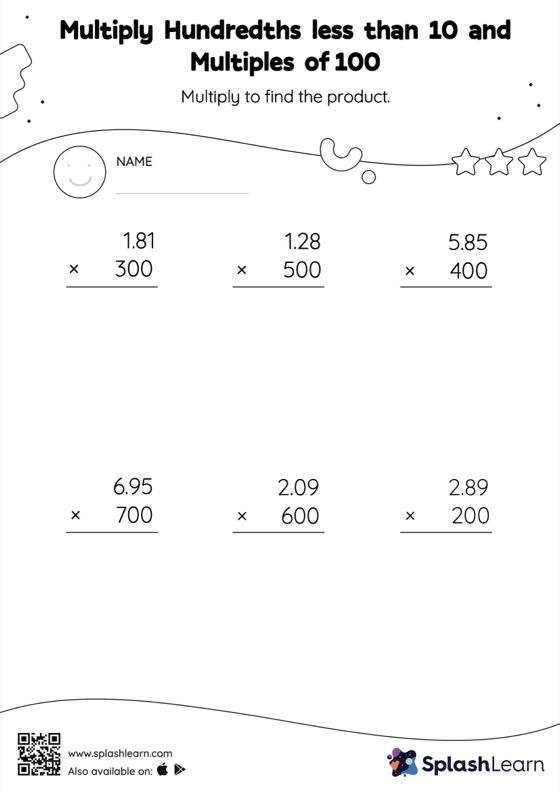# Multiply Hundredths less than 10 and Multiples of 100: Vertical Multiplication Worksheet

Home > Multiply Hundredths less than 10 and Multiples of 100: Vertical MultiplicationThis multiply hundredths less than 10 and multiples of 100 worksheet consists of a set of questions on multiplication, which help students develop fluency. In this worksheet, students practice solving problems using the column method. This method is especially helpful with problems involving bigger multi-digit numbers as the format provides an easy structure to follow.